The seemingly complex calculations of flight make the concept of “pilot math” sound vaguely terrifying. Prospective student pilots wonder, “What kind of math do pilots use?” and “Do I have what it takes to handle pilot math?”

Before you get discouraged and give up on the idea, yes, math and physics are important subjects that pilots must be competent in, but it often is not nearly as complex as you may think. In most cases, there are formulas to guide you through the computations as well as software and devices that can run the numbers for you. Once you understand the concepts behind the equations, the actual math itself is not so challenging. Simply choose the correct formula, plug in your numbers, and arrive at the answer.Ready to learn what it takes and conquer your fear of pilot math? Here is what you need to know:

## What types of math do pilots?

There are several types of math that pilots need to familiarize themselves with and be able to apply. Basic arithmetic, geometry, trigonometry, interpolation, and mental math are all part of being a pilot.

### Basic arithmetic

Basic arithmetic is the first kind of math you learned back in elementary school: addition, subtraction, multiplication, and division. For most of us, basic arithmetic comes easily and is not overly intimidating. Since this is the type of pilot math you are likely to be doing manually or mentally at times and not always with a calculator, be sure to pay attention to detail and avoid making careless mistakes.

### Geometry

Geometry focuses on shapes, sizes, and spatial relationships between objects, making it a very valuable branch of mathematics for aviation. Formulas are prevalent in geometry, so it helps to memorize the most common and have cheat sheets or reference materials for others.

### Trigonometry

The mathematical branch of trigonometry focuses on relationships between the sides and angles within a triangle. One of the most common ways that pilots apply trigonometry is when they are making wind correction or crosswind component calculations. The good news is that pilots do not need to be experts in trigonometry to do these calculations. They simply need to understand how to do the calculations, often using an E6B or a flight calculator app.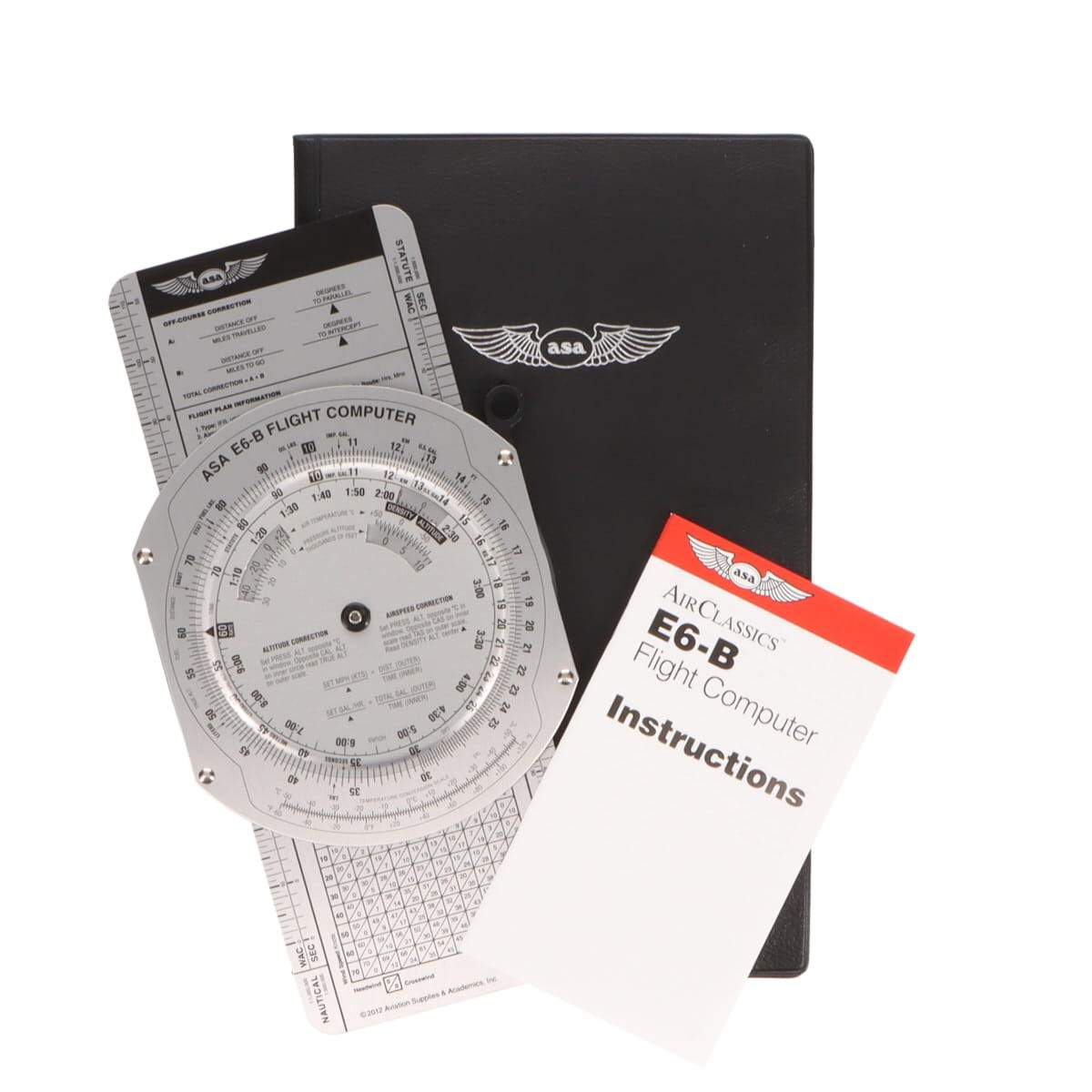### Interpolation

Interpolation is the mathematical term for making an educated estimation based on surrounding data. This technique uses basic arithmetic skills as well as algebraic skills since the unknown variable you are solving for, “x,” must be determined based on its relationship to the data you do have.

### Mental Math

Although pilots use their physical calculators and calculator apps to do much of the work for them, there are times when you must perform quick and accurate math in your head. Mental math is a learned skill and one that should be practiced. Start improving your skills by reading Mental Math for Pilots.## What do pilots need to use math for?

Principles of flight, weight and balance, fuel needs, general navigation, flight planning and monitoring, descent planning, crosswind components, and more all require the use of pilot math. Here are just a few real-world examples of how pilots use math on a regular basis:

### Weight calculations

Weight calculations use the basic arithmetic skills of addition and subtraction. For an airplane to fly, it cannot weigh more than the amount of lift it generates. Each plane has a known empty weight and everything that is brought onboard including fuel, passengers, luggage, and cargo must be added to this empty weight. The final number must be at or below the maximum takeoff weight established by the airplane manufacturer and listed in the pilot’s operating handbook (POH).

For example, a Cessna 180K has an empty weight of 1,650 pounds and a maximum takeoff weight of 2,810 pounds. To calculate the useful load, or the amount of weight that can be added to the plane, a pilot will subtract the empty weight from the maximum takeoff weight:

Maximum takeoff weight – empty weight = useful load

In our example this comes out to: 2,810-pound takeoff weight – 1,650 empty weight = 1,160 pounds useful load.Now that we know that a maximum of 1,160 pounds of useful load can be added to the plane, we will weigh everything that gets onboard the aircraft (ourselves included) and subtract those weights from the useful load to ensure we are still below that max threshold.

### Fuel calculations

Fuel calculations also use basic addition and subtraction skills. Take the same Cessna 180K and assume we need to know how many pounds of our available 1,160-pound useful load must be allocated for fuel. If we average a fuel burn of 13 gallons per hour and our destination is 2 hours away, we multiply our per gallon burn by the number of hours we will be traveling:

Per hour fuel burn x number of hours = Gallons of fuel needed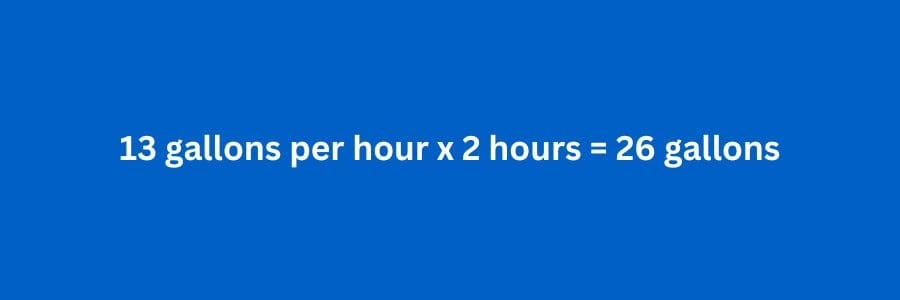Since in aviation, fuel load is calculated in pounds, we will convert our 26 gallons into pounds again using multiplication and the known variable of each gallon of Avgas weighing 6 pounds.

Gallons of fuel needed x weight per gallon = Fuel weight

Based on this equation we have: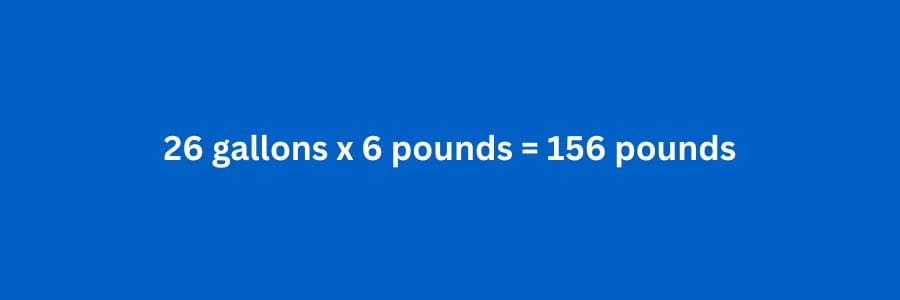Our pilot math tells us that we will need a minimum of 156 pounds of fuel to get us to our destination, but we are not done yet. The FAR requirements state that in addition to our minimum fuel budget, we will also need to add enough fuel to get us to the most distant alternate destination on the flight plan as well as a 45-minute reserve.

If the alternate destination adds an additional 15 minutes of flight time, we can use our basic arithmetic skills to add up the total amount of additional flight time we need to budget for.

Additional flight time to alternate destination + fuel reserve time = Total additional flight time

For our example this looks like:We know that our fuel burn is 13 gallons per hour, so for an extra hour of flight time, we will need to load an additional 13 gallons of fuel. Again, multiply the gallons of fuel by the known weight per gallon to calculate the total weight of the extra fuel.

Gallons of fuel x weight per gallon = Extra fuel weightThe final step in our fuel calculation is to add the initial minimum fuel weight to our extra fuel weight so we have a total weight for the amount of fuel we need to take on and account for in our max weight calculation.

Minimum fuel weight + extra fuel weight = Total fuel weight

For our example plane we have: 156 pounds minimum fuel + 78 pounds extra fuel = 234 total pounds of fuel needed.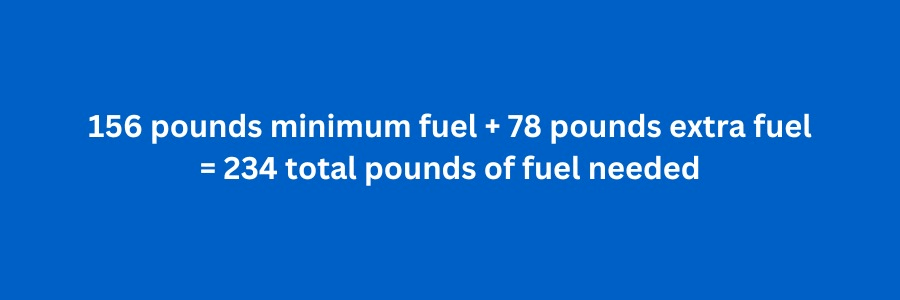### Crosswind component calculations

When you are landing or taking off, if there is a wind which is perpendicular to the runway, this is referred to as a crosswind. A crosswind alters the aerodynamics of your aircraft and makes landings and takeoffs more difficult. Too strong a crosswind can be dangerous, so it is important to calculate the crosswind and determine if it is safe to takeoff or land under those conditions and with your skillsets. If you proceed with the maneuver, you will also be using the crosswind component calculation to help adjust and compensate for the crosswind.

Precise crosswind component calculations are done using trigonometric equations, so you will need your calculator. The basic formula is:

Crosswind component = Wind speed x cosine of angle between the wind and runway

Of course with crosswind components as with many other types of pilot math, pilots have developed rules of thumb that can serve as a ballpark estimate if you do not have the calculator to do the precise equation or you want to check your work.

In this case, pilots remember that if the angle between the wind and runway is 15 degrees, the crosswind component is about 25% of the wind velocity. At 30 degrees it is 50%, at 45 degrees it is 75%, and at 60+ degrees, the crosswind component is 100% of wind speed.

While rules of thumb like this should not be used where absolute precision is needed, they can help augment pilot math and serve as a cross check. For example, if you were planning your takeoff and knew that there was a 40 knot wind at a 30 degree angle to the runway, based on your rules of thumb, you would expect your calculator to tell you that the crosswind component is around 20 knots. If the calculator read 18 knots, you would believe it to be accurate because it is close to the rule of thumb estimate. If for some reason, your calculated crosswind component read double the estimate at 40 knots, that would be a flag and you would know to review your calculations.

### Descent Rate calculations

For pilots to land safely, they need to hold to a given descent angle. To do this, they must calculate how far out from the airport they should begin the descent and how rapid that descent should be so that they maintain the proper descent angle and stay on the glidepath.

To understand descent rate math, visualize the ground as the base of a triangle, with a perpendicular invisible vertical line from the ground to the aircraft as one side of  the triangle, and a diagonal line from the aircraft to the ground as the other side of the triangle. The angle between the diagonal line and the ground is the descent angle. For this example, we will say that we need that angle to be 3-degrees.

Now we must determine how fast we need to lose altitude to keep that 3-degree glidepath angle as we approach the runway at a given speed. To do this we need to use the formula:

Tangent of glidepath angle = altitude loss necessary per minute / groundspeed per minute

Several data conversions are necessary because groundspeed is measured in nautical miles per hour whereas we want to solve for our descent rate which is expressed in feet per minute. To start our conversions, we first use the formula:

Groundspeed in nautical miles per hour / 60 = Groundspeed in nautical miles per minute

If our groundspeed is 100 kts and we divide that by 60, we get a speed of approximately 1.66 nautical miles per minute. Now we need to convert this to feet per minute.

Groundspeed in nautical miles per minute x 6,080 feet per mile = Groundspeed in feet per minuteNow that our groundspeed is expressed in feet per minute, we are ready to calculate the rate of descent we need to stay on glidepath.

Descent rate = Groundspeed per minute x tangent of glidepath angle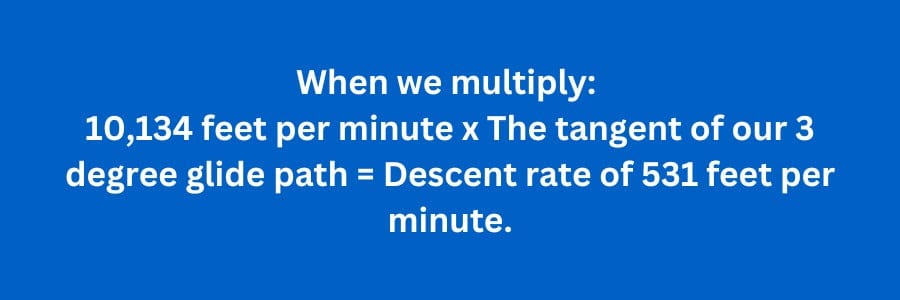This may sound complicated, but usually if you do the equation, you will use a calculator. Also, since 3-degrees is a common glideslope angle, pilots have worked out another rule of thumb that gets you close if you do not have a calculator to plug your equations into. Simply take your groundspeed in knots and multiply it by 5 to arrive at an approximate descent rate of 500 feet per minute which is very close to the precisely calculated 531 feet per minute you get when using the equation.

## Takeaways

Pilot math can seem intimidating, but the important thing to remember is that if you understand the purpose and theory behind the equations, following the math itself often gets easier. Also, in many cases you can simply use a calculator or apply a rule of thumb estimation to arrive at a quick ballpark answer to a math question that does not require specificity.

If being a pilot is your dream, do not let the math scare you away. Instead invest some time in learning more about how pilots use math. To get you started, Aviation-Press Everything Explained for the Professional Pilot 14th Edition
is an excellent reference book contains simple formulas along with rules of thumb, tips, and strategies for mathematical success.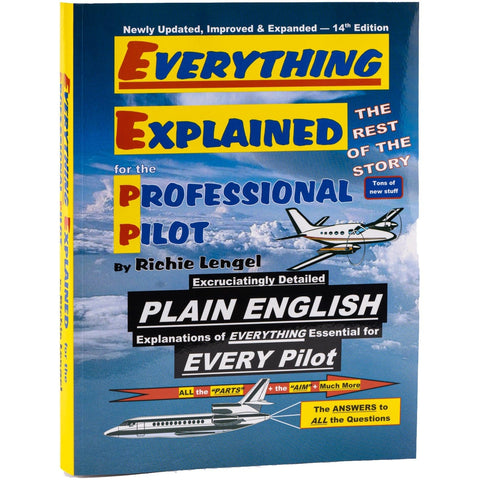All comments are moderated before being published

Featured productsHigh Flying Models
💰 Save 25% Today
Cessna® 172 Skyhawk SP (Red) Clear Canopy Limited Edition Large Mahogany Model
Sale price\$336.75 Regular price\$449.00
In stockBose
Online Rebate
Bose A30 Aviation Headset with Bluetooth
Sale price\$1,299.00
6 In stock

## Latest Blog Posts

View all## Space Force: Understanding the Newest Branch of the Military

Military
We’ve all heard that the Space Force logo looks like Star Trek and Space Force personal are going to be “guardians” of the galaxy (or at least the near-Earth portion of it), but what else do you know about the sixth and newest branch of the United States military?## Aviation Alphabet: Learn the Phonetic Alphabet for Aviation (A to Z)

Was that an “m” or an “n?” Even today most of us have experienced the frustration of trying to communicate the spelling of our name, a street name, or other information over the phone only to have the other party not understand it. Now imagine living back in the early days of telephone when the connection was not remotely as good and the audio nowhere near as crisp as it is today.## Ground Reference Maneuvers: How to Ace Your Checkride

Maneuver
One of the final steps in become a full-fledged pilot is to pass your checkride. To do that, among other things, you need to nail your ground reference maneuvers.